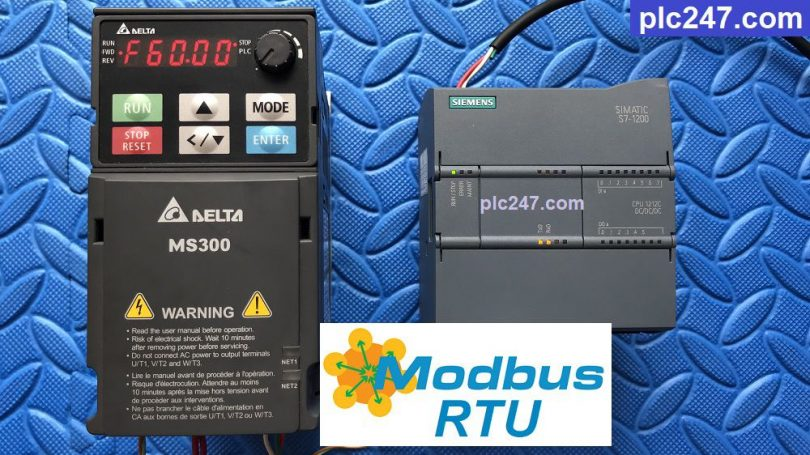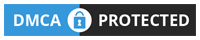# Siemens S7 1200 “Modbus RTU” DELTA MS300 VFDWritten by

PLC Siemens S7-1200 is a type of PLC that is extremely commonly used in factories around the world. Today plc247.com will guide you to use it to control DELTA MS300 inverter by Modbus-RTU.

I use Expansion Module S7-1200 CB 1241 (or S7-1200 CM1241 also) to control Modbus-RTU RS485.

#### DELTA MS300 Modbus Configuration

We need to set the minimum communication parameters as follows:

+ P00.20 = 1 (Set Frequency via Modbus)

+ P00.21 = 2 (Control Motor via Modbus)

+ P09.00 = 1 (Address = 1)

+ P09.01 = 9.6 (9600bps)

+ P09.02 = 0

+ P09.03 = 1.0 (Time Err Communication = 1.0s)

+ P09.04 = 14 (Choose Modbus-RTU 8bit/Even/1)

+ Motor Control Address = 40001 (dec) + 2000 (hex) = 40001 + 8192 (dec)
= 48193 (dec)

• Set Word 48193 = 18 >>> Forward Run
• Set Word 48193 = 34 >>> Reverse Run
• Set Word 48193 = 1 >>> Stop Run

+ Frequency Address = 40001 (dec) +20001 (hex) = 40001 + 8193 (dec)
= 48194 (dec)

##### Resigter for Data Monitoring
• Output Frequency = 40001(dec) + 2103(hex) = 40001 + 8451 = 48452 (dec)
• Output Current = 48453 (dec)
• DC-BUS Voltage = 48454 (dec)
• Output Voltage = 48455 (dec)

#### S7-1200 Communication Setting

Configuration Module S7-1200 CB1241

Create Data Types and Data Blocks

### Siemens S7-1200 PLC Programming

+ Frequency Set Command

+ Control Motor Command

### Detailed Installation Instruction Video

======

Required software and documentation

+ TIA Portal V15.1 for PLC Siemens Programming